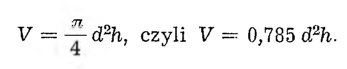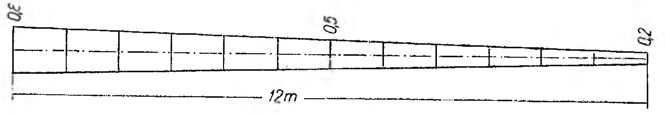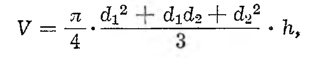# A strange tree trunkIn forestry practice, the volume of a fallen tree trunk is calculated in this way, as if it were a rotating cylinder, the diameter of the cylinder is measured at half the length of the stump. So if we denote the length of a tree trunk as h, and the diameter in the middle of the length will be d, then the volume of such a trunk is calculated by the formula:Usually it is taken as an approximation: V = 0,8 d²h.
Here was a 12-meter-long tree trunk. He had a trunk at the thicker end 0,8 m in diameter, and on the thinner end 0,2 m in diameter. The trunk was evenly tapered, as shown in the picture.The customer has come, who wanted to buy this trunk and asked to calculate its volume.

It's not hard to see, that the diameter of the trunk at half its length was 0,5 m. Hence the volume of the trunk:

V = 0,8 • 0,52 • 12 = 2,4 m³.

The amount due was calculated counting after 100 PLN cubic meter; fell out 240 PLN. But at this point, the client changed his mind. He said, that he actually needs a trunk of 10 meters in length. So it was cut off from the thin end 2 m of the trunk. The rest of the trunk was h - 10 m, and the half-length diameter was d - 0,55 m. A new volume was calculated, which had to be smaller. It fell out of the calculation:

V = 0,8 • 0,552 • 10 = 2,42 m³.

At the same price 100 PLN per cubic meter trunk shortened by 2 m cost 242 PLN, that is, Fr. 2 PLN more than the primary stock.

And yet there was still a length of the trunk 2 m, of average diameter 0,25 m. The volume of this segment is

V = 0,8 • 0,252 • 2 = 0,1 m³,

so only this episode cost 10 PLN. Where did these strange things come from?

Well, the formula used to calculate the volume of the tree trunk is to be found. The pattern is inaccurate. To calculate more accurately the volume of the tree trunk, the formula should be usedwhere d1 and d2 denote the diameter of the stem at both ends, and h is the length of the trunk. According to this formula, the entire trunk cost 263,76 PLN, shortened trunk 253,82 PLN, a stretch of the trunk 9,94 PLN.